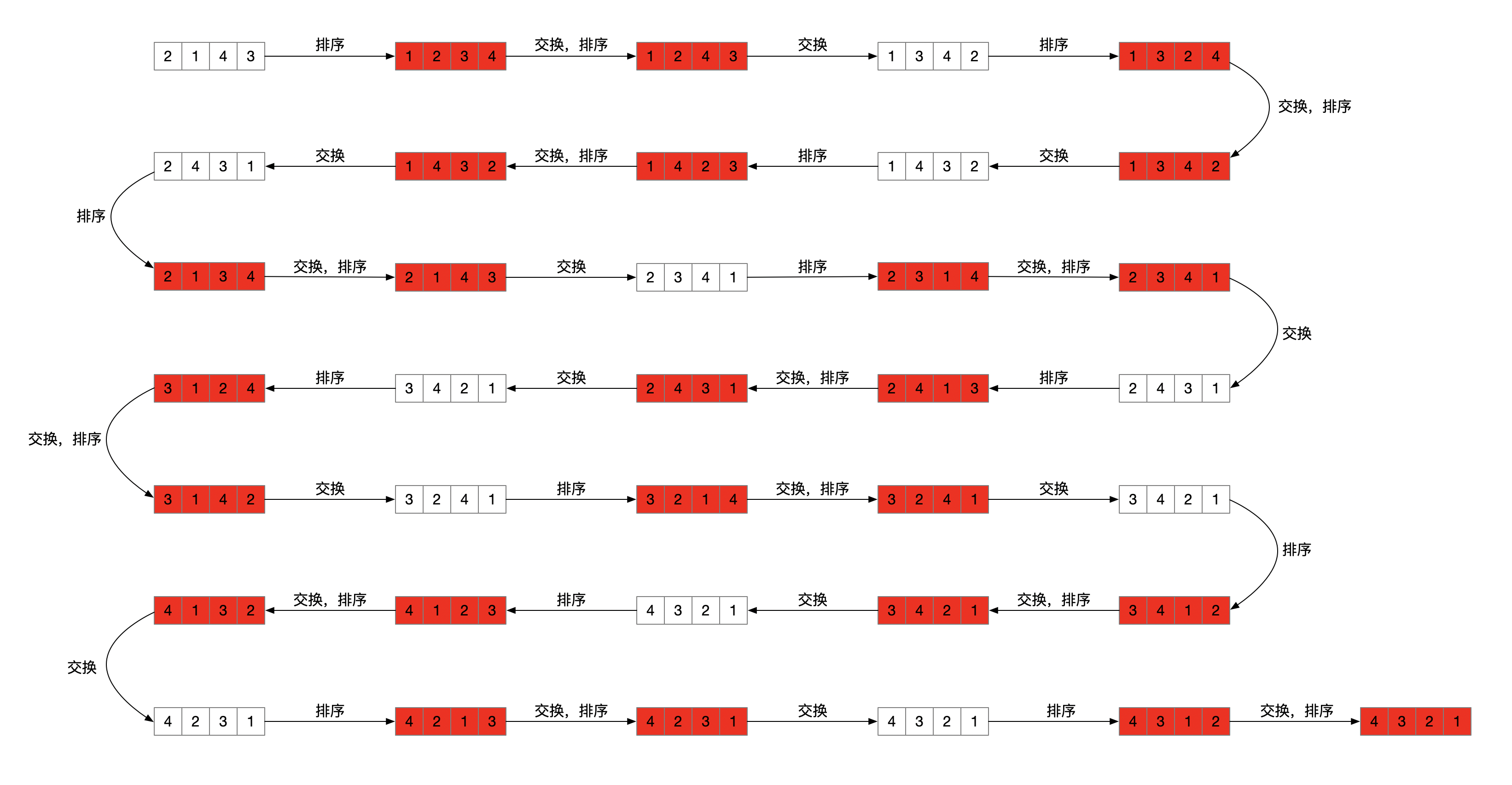# 数组全排列算法非递归实现

1、arr先进行排序，从小到大，排序后{1, 2, 3}

2、从右往左，找到第一个i，使得arr[i] < arr[i+1]，那么在arr[i+1:len]里找出一个最小的arr[min] > arr[i]，然后交换arr[i]和arr[min]，最后从小到大排序arr[i+1:len]如此反复

1、arr从小到大排序，得到排列[1, 2, 3]

2、arr = 3 > arr = 2，arr[2:3] = 3，因此直接交换arr和arr，变成了[1, 3, 2]，而arr[2:3]排序也只有一个2，因此得到排列[1, 3, 2]

3、arr = 3 > arr = 1，arr[1:3] = [3, 2]，arr[min] = arr = 2， 因此交换arr和arr，变成了[2, 3, 1]，最后arr[1:3]从小到大排序，得到排列[2, 1, 3]

4、arr = 3 > arr = 1，arr[2:3] = 3，因此直接交换arr和arr，变成了[2, 3, 1]，而arr[2:3]排序也只有一个1，因此得到排列[2, 3, 1]

5、arr = 3 > arr = 2，arr[1:3] = [3, 1]，arr[min] = arr = 3，因此交换arr和arr，变成了[3, 2, 1]，最后arr[1:3]从小到大排序，得到排列[3, 1, 2]

6、arr = 2 > arr = 1，arr[2:3] = 2，因此直接交换arr和arr，变成了[3, 2, 1]，而arr[2:3]排序也只有一个1，因此得到排列[3, 2, 1]

1、首先从小到大排序，能够保证第一个排列是最小的一个排列

2、arr[i]逐渐变大，arr[i+1, len]不停增大，arr[0:i]保持不变`package com.lihuia.bean;import java.util.ArrayList;import java.util.Arrays;import java.util.List;/** * Copyright (C), 2018-2020 * FileName: Permutation * Author:   lihui * Date:     2020/1/3 */public class Permutation {    private List<String> list = new ArrayList<>();    public void swap(int[] nums, int i, int j) {        int temp = nums[i];        nums[i] = nums[j];        nums[j] = temp;    }    public void quickSort(int[] nums, int start, int end) {        int left = start;        int right = end;        int temp;        if (left <= right) {            temp = nums[left];            while (left != right) {                while (left < right && nums[right] >= temp) {                    right--;                }                nums[left] = nums[right];                while (left < right && nums[left] <= temp) {                    left++;                }                nums[right] = nums[left];            }            nums[right] = temp;            quickSort(nums, start, left - 1);            quickSort(nums, right + 1, end);        }    }        public String toString(int[] nums) {        return Arrays.toString(nums);    }    public void permuteUnique(int[] nums) {        int len = nums.length;        if (len <= 1) {            list.add(toString(nums));            return;        }                quickSort(nums, 0, len - 1);        list.add(toString(nums));                while (true) {            int right = len - 1;            int i;            int min = 0;            for (i = right - 1; i >= 0; i--) {                if (nums[i] < nums[i + 1]) {                    min = i;                    break;                } else if (i == 0) {                    return;                }            }            for (i = right; i >= 0; i--) {                if (nums[i] > nums[min]) {                    break;                }            }            swap(nums, min, i);            quickSort(nums, min + 1, right);            list.add(toString(nums));        }    }    public String getPermute(int[] nums, int index) {        permuteUnique(nums);        System.out.println(list.size());        return list.get(index);    }}`

OVER